# Are there any points other than the origin where cosines are totally in phase?

• B
PainterGuy
TL;DR Summary
This question just came to my mind and I thought I should confirm it. Are the cosine functions with the same amplitude but different frequencies are totally in phase at any other point other than the origin? The frequency, f, could be 0<f<∞. I'm not sure if the answer is obvious.
Hi,

This question just came to my mind and I thought I should confirm it. Are the cosine functions with the same amplitude but different frequencies are totally in phase at any other point other than the origin? The frequency, f, could be anywhere between 0 < f < ∞. I'm sorry if the answer is too obvious.

I have graphed below some cosines below and to me it looks that the origin is only point where they are totally in phase.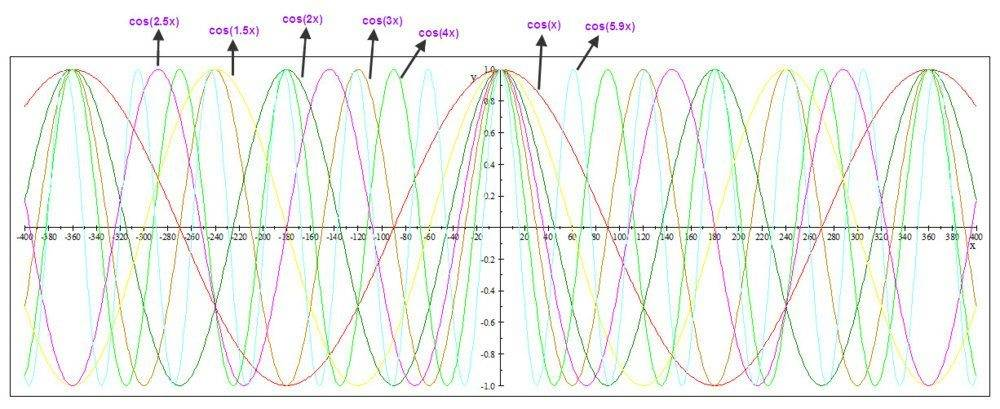#### Attachments

Mentor
No. At point x=x0 you have ##\cos(\pi \frac x {x_0} ) = -1## but ##\cos(2\pi \frac x {x_0} ) = 1##.

This doesn't mean x=0 is special - it just follows from the collection of functions you consider. If you consider the set cos(c(x-2)) for different c then they will all line up at x=2.

•PainterGuy
PainterGuy
Thank you for confirming that the cosine functions with the same amplitude but different frequencies are only in phase at the origin.

I presume you mean only in one cycle of cos(x).

PainterGuy
Thanks!

I presume you mean only in one cycle of cos(x).

No, not just one cycle. I'm considering all the cycles from -∞ to ∞.

The frequency, f, is a real number and could be anywhere between 0 < f < ∞.

Edit:
I understand that it's said that a sine or cosine functions are periodic over 2π but it doesn't look like it holds when frequencies are non-integer. I have tried to illustrate my point below.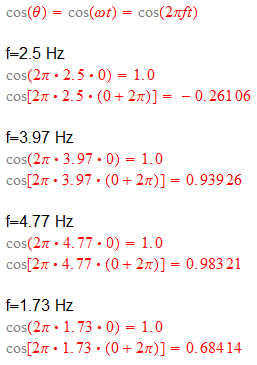Last edited:
Mentor
The cosine function - that is one single function - has a period of 2 pi.

fc(x)=cos(cx) has a period of 2pi/c, but that is a different function for each c.

•PainterGuy
PainterGuy
Thanks.

I'm not sure why @mathman said, "I presume you mean in only one cycle of cos(x)". Because the
the cosine/sine functions with the same amplitude but different frequencies are only in phase at the origin; in other words nowhere other than the origin, i.e. t=0, their peaks or troughs ever match up anywhere over all their cycles where -∞ < t < ∞.

Last edited:
Gold Member
He said it because two cosines whose frequencies are related by a rational ratio will be in phase an infinite number of times.

•PainterGuy and DaveE
PainterGuy
Hi again,

Suppose at π radians the phase of each cosine is measured in relation to cos{2π(1)t} where 0 < f < ∞ and -∞ < t < ∞. It would give you a set of phases for all cosines at π radians with respect to f=1 cosine. You could use any point other than π radians. Now the question is if we would get the same set of phase relationships at any other point along the t axis.

I understand that cosine is an even function and symmetric along y-axis but, for example, if you check, cos(5.9x), https://imagizer.imageshack.com/img922/5073/b2qFGJ.jpg , you can see that the phase differs for -80°, 80°, -360°, 360°, and so on. If on one side curve is going up, on the other side it is going down. It's really confusing. Where am I going wrong?

Note to self: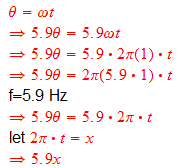Mentor
If on one side curve is going up, on the other side it is going down.
That's exactly what you get from mirror symmetry. Both go up/down together if you "go away from zero".

PainterGuy
Thank you.

That's exactly what you get from mirror symmetry. Both go up/down together if you "go away from zero".

But they are not going up/down together. One is going up and the other, the symmetric point, is going down. Please check the plot in my previous post.

Could you please also comment on my other question? Thanks.

Mentor
I don't understand your other question.
One is going up and the other, the symmetric point, is going down.
That depends on the direction you look at.

Here is a black shape that is clearly mirrored at the red line. Call "up" and "down" what you want.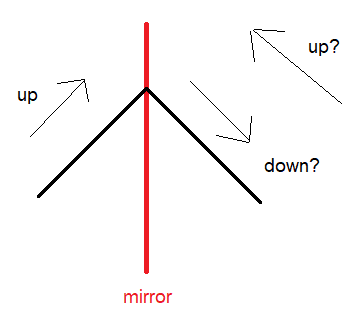•PainterGuy
PainterGuy
Thank you!

Here is a black shape that is clearly mirrored at the red line. Call "up" and "down" what you want.

I didn't phrase my question properly because now I see that it was something else, a related point, which was actually troubling me. I believe that in case of sinusoids two points are considered to be symmetric when their amplitudes and phases are equal. If one point is going up and the other down, the phases are not going to be equal. For example, in this plot, https://imagizer.imageshack.com/img924/9855/rRjJ3A.jpg, you can see that each set of points have equal amplitudes but, as I said earlier, one is going up and the other down which implies different phases.

Let me rephrase the other question. In the picture below, the phase of each cosine is measured at π radians or 180°; the blue line shows the point where the phase measurement of cosines is taking place. For example, the phase of cos(x) is 180°, phase of cos(2x) is 0°, the phase of cos(2.5x) is approximately 90°, phase of cos(3x) is 180°, and so on. Assuming that we are able to measure phase of each cosine for 0 < f < ∞. It would give us a set of phases at t=π radians like this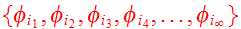. My question is as the blue line sweeps through the t axis (or, x-axis) from 0 < t < ∞, would we get the same set(s) of phases at some other point(s)?

By the way, I haven't included negative side of x-axis because one might say as the cosine is symmetric therefore you would also get the same set of phases at t=-π although I'm confused about this point as my starting statement in this post shows.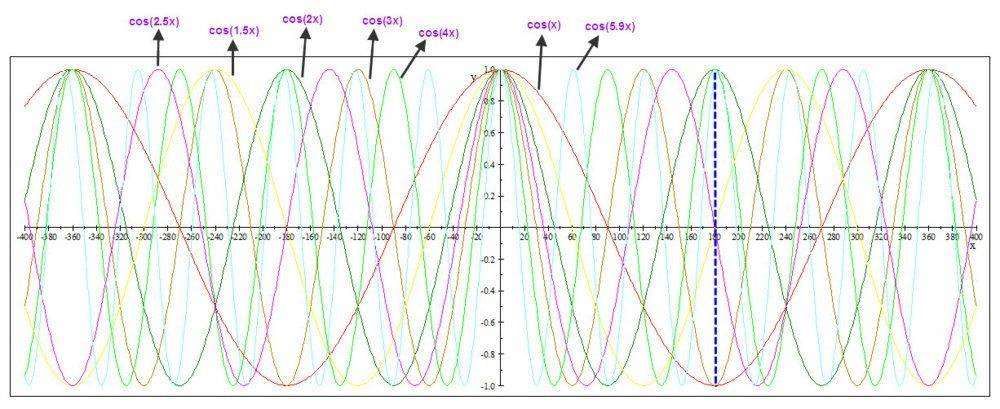#### Attachments

Mentor
I believe that in case of sinusoids two points are considered to be symmetric when their amplitudes and phases are equal.
That is wrong.
My question is as the blue line sweeps through the t axis (or, x-axis) from 0 < t < ∞, would we get the same set(s) of phases at some other point(s)?
Never for all functions you consider. This is a generalization of the example I gave before. In particular, cos(x) and cos(pi x) do not have any pair of points where both functions have the same phase in both points. Proof: Let's assume there would be two such points, x1 and x2. Having the same phase means a phase difference of 2 pi n for some integer n. That means there are integers ka, kb such that x2-x1 = 2 pi ka (same phase of the cos(x) function) and pi (x2-x1) = 2 pi kb or simplified x2-x1 = 2 kb (the same phase for the cos(pi x) function). Combining both equations we get pi = kb/ka, as ratio of integers pi would have to be a rational number. It isn't.

•PainterGuy
PainterGuy
Thank you!

So, I was wrong about my definition of two symmetric points of a sinusoid. The symmetric points should have the same amplitudes but phases could be different. For example, if one point has an amplitude of +5 and going up then the other symmetric point should also have an amplitude of +5 and could be going up/down.

But I think I haven't still been able to convey my other query to you properly. Let me try again. I'm not saying that if the phases are going to be the same for two or more, or for all the cosines where 0 < f < ∞. I was trying to say the following. Suppose that at t=π, the phase of first cosine is 'a', the phase of second cosine is 'b', the phase of third cosine is 'c', and so on. The phases of all cosines at t=π constitute a set S={a, b, c, ...}. My question is if there could be another value(s) of 't' at which the resulting set of phases is exactly the same as S.

Previously, it was concluded that only at t=0 the phases of all cosines could be same.

Thanks a lot.

Mentor
But I think I haven't still been able to convey my other query to you properly. Let me try again. I'm not saying that if the phases are going to be the same for two or more, or for all the cosines where 0 < f < ∞. I was trying to say the following. Suppose that at t=π, the phase of first cosine is 'a', the phase of second cosine is 'b', the phase of third cosine is 'c', and so on. The phases of all cosines at t=π constitute a set S={a, b, c, ...}. My question is if there could be another value(s) of 't' at which the resulting set of phases is exactly the same as S.
For specific, fixed sets of functions this can be possible, but there are functions where this is not the case, and I gave an example of a set of two functions.

•PainterGuy
PainterGuy
Thank you!

For specific, fixed sets of functions this can be possible, but there are functions where this is not the case, and I gave an example of a set of two functions.

Never for all functions you consider. This is a generalization of the example I gave before. In particular, cos(x) and cos(pi x) do not have any pair of points where both functions have the same phase in both points. Proof: Let's assume there would be two such points, x1 and x2. Having the same phase means a phase difference of 2 pi n for some integer n. That means there are integers ka, kb such that x2-x1 = 2 pi ka (same phase of the cos(x) function) and pi (x2-x1) = 2 pi kb or simplified x2-x1 = 2 kb (the same phase for the cos(pi x) function). Combining both equations we get pi = kb/ka, as ratio of integers pi would have to be a rational number. It isn't.

I understand it now.

Note to self:
Never for all functions you consider. This is a generalization of the example I gave before. In particular, cos(x) and cos(pi x) do not have any pair of points where both functions have the same phases in both points. Proof: Let's assume there would be two such points, x1 and x2; it means that at x1 cos(x) has phase φ₁ and cos(pi x) has phase φ₂ therefore at x2 cos(x) has once again phase φ₁ and in the same manner cos(pi x) has phase φ₂. Having the same phase means a phase difference of 2 pi n for some integer n. That means there are integers ka, kb such that x2-x1 = 2 pi ka (same phase of the cos(x) function) and pi (x2-x1) = 2 pi kb or simplified x2-x1 = 2 kb (the same phase for the cos(pi x) function). Combining both equations we get pi = kb/ka, as ratio of integers pi would have to be a rational number. It isn't.

When you have 0 < f < ∞, this would definitely be true that as the blue line sweeps through the t axis (or, x-axis) from 0 < t < ∞, there wouldn't be the same set(s) of phases at some other point(s).